Courses

# Reactions Involving Gaseous Species Civil Engineering (CE) Notes | EduRev

## Civil Engineering (CE) : Reactions Involving Gaseous Species Civil Engineering (CE) Notes | EduRev

The document Reactions Involving Gaseous Species Civil Engineering (CE) Notes | EduRev is a part of the Civil Engineering (CE) Course Thermodynamics.
All you need of Civil Engineering (CE) at this link: Civil Engineering (CE)

Reactions involving gaseous species

We now consider eqn. 8.50 that represents a relation that connects equilibrium composition with the equilibrium constant for a reaction. The activities aˆi in eqn. 8.50 contains the standard state fugacity of each species which – as described in section 8.1 – is chosen as that of pure species at 1 bar pressure. The assumption of such a standard state is necessarily arbitrary, and any other standard state may be chosen. But the specific assumption of 1 bar pressure is convenient from the point of calculations.  Obviously the value of the state Gibbs free energy Gio of the species needs to correspond to that at the standard state fugacity. In the development that follows we first consider the case of reactions where all the species are gaseous; the case of liquids and solids as reactants are considered following that. For a gas the standard state is the ideal-gas state of pure i at a pressure of 1 bar. Since a gaseous species at such a pressure is considered to be in an ideal gas state its fugacity is equal to its pressure; hence at the standard state assumed at the present, fi o = 1 bar for each species of a gas-phase reaction. Thus, the activity and hence eqn. 8.50 may be re-written as follows: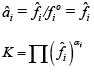For the use of eqn. 8.57, the fugacity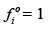must be specified in bar [or (atm)] because each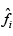is implicitly divided byo 1 bar [or 1(atm)]. It follows that the equilibrium constant Kis dimensionless.This is true also for the case of liquid and/or solid reactive species, though, as is shown later, the standard state fugacity is not necessarily 1 bar, since for condensed phases the fugacity and pressure need not be identical at low pressures.

By eqn. 6.129, for gaseous species,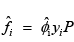Thus eqn. 8.57 may be rewritten as: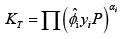...(8.58)

On further expanding the above equation: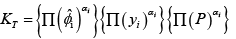...(8.59)

OR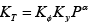...(8.60)

Where: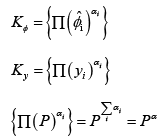...(8.61 to 8.63)

An alternate from of eqn. 8.60 is: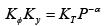...(8.64)

Both the terms Kφ and Ky contain the mole fraction yi of each species. As given by eqn. 8.29 or 8.39, all the mole fractions may be expressed as a function of the reaction co-ordinate ξ of the reaction(s). Hence, for a reaction under equilibrium at a given temperature and pressure the only unknown in eqn. 8.64 is the equilibrium reaction co-ordinate ξe . An appropriate model for the fugacity coefficient (based on an EOS: virial, cubic, etc.) may be assumed depending on the pressure, and eqn. 8.64 may then solved using suitable algorithms to yield the equilibrium mole fractions of each species. A relatively simple equation ensues in the event the reaction gas mixture is assumed to be ideal; whence
φˆi = 1. Thus, eqn. 8.64 simplifies to: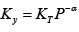...(8.65)

or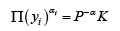...(8.66)

Yet another simplified version of eqn. 8.64 results on assuming ideal solution behavior for which (by eqn.7.84):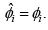Thus: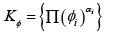...(8.67)

This simplification renders the parameter Kφ independent of composition. Once again a suitable model for fugacity coefficient (using an EOS) may be used for computing each φ i and eqn. 8.64 solved for the equilibrium conversion.

Offer running on EduRev: Apply code STAYHOME200 to get INR 200 off on our premium plan EduRev Infinity!

## Thermodynamics

56 videos|92 docs|33 tests

,

,

,

,

,

,

,

,

,

,

,

,

,

,

,

,

,

,

,

,

,

;